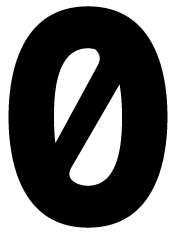# Best Practices with nil in Objective-C

## Unnecessary to give nil as initial value```NSString *myString = nil;
```

is the same thing without nil, so just keep it off:

```NSString *myString;
```

The same goes with properties. Remember that nil is equivalent to 0 which is equivalent to NO for a BOOL type. So no need to do this:

```@property (nonatomic, assign) BOOL didLoad;
...
```

## Delegate

```if (self.delegate) {
if ([self.delegate respondsToSelector:@selector(@"didFinish")]) {
[self.delegate didFinish];
}
}
```

This can be simplified, since if the delegate is nil, nil can receive messages which will evaluate to nil, so here it is simplified:

```if ([self.delegate respondsToSelector:@selector(@"didFinish")]) {
[self.delegate didFinish];
}

```

## Simplify conditionals

```if (myObj == nil)
```

can be simplified to

```if (!myObj)
```

Also avoid the !=:

```if (myObj != nil)
```

this is hard to read and look how simple this is:

```if (myObj)
```

## Remember that anywhere along the line of objects can be nil

```if (a.b.c)
```

So this will evaluate to false if a, b, or c are nil. This is perfectly ok, so no need to do this:

```if (a)
if (a.b)
if (a.b.c)
```

## Just let things be nil

I've noticed a lot of code in the past where I'd set an array to be empty: `@[]` rather than just letting the array remain nil, or uninitialized. It's ok to let things be nil. If you have an array object that is nil, checking its count has the same behavior as if it was an empty array. For example, the following conditional will evaluate as true for both myArray = nil and myArray = @[]

```if (myArray.count == 0)
```

Date: 2014-11-17T15:36+0000

Org version 7.9.3f with Emacs version 24

Validate XHTML 1.0